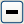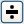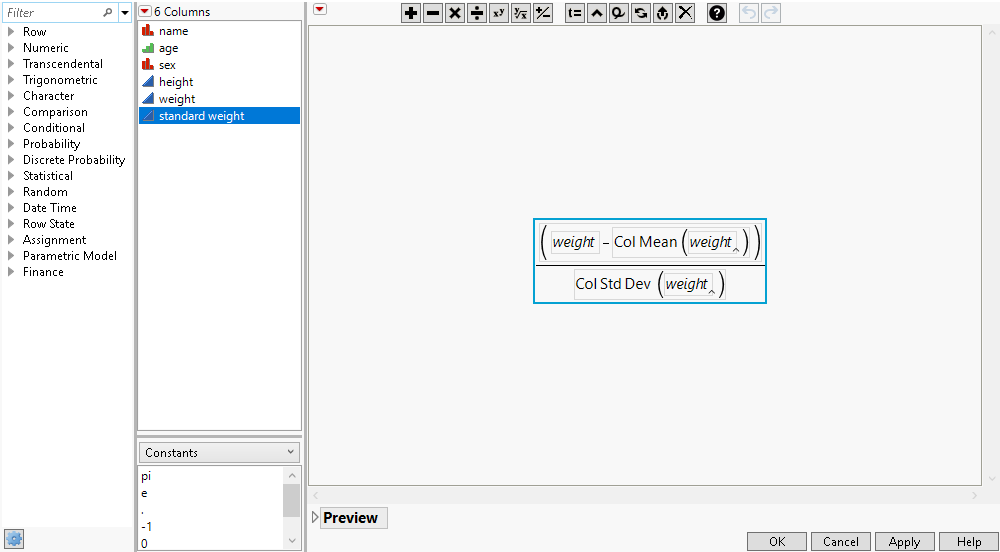Publication date: 05/24/2021

## Use Basic Features in the JMP Formula Editor

The following example uses the Big Class.jmp sample data table to walk you through using the basic features of the Formula Editor. You can find Big Class.jmp in the sample data folder, which was installed when you installed JMP.

Big Class.jmp has a column called weight. Suppose you want a new column that computes standardized weight values.

To create this column using a formula to obtain its values, follow these steps:

1. Select Help > Sample Data Library and open Big Class.jmp.

2. Select Cols > New Columns.

3. Type the new name, standard weight, in the box beside Column Name.

4. Select Formula from the Column Properties menu.

5. Click the empty formula element in the formula editing area to select it.

When you create a formula and Show Boxing is checked, the selected portion of the formula is outlined with a thin blue line. See Hide and Show Outlines Formulas. All terms within the smallest nesting box relative to the place that you clicked become selected, and the subsequent action applies to those combined elements.

Next, enter the formula that standardizes the weight values.

1. While the initial missing term is selected, click weight in the Columns list.

2. Click the minus buttonin the Formula Editor keypad.

A new missing term appears after the minus sign as shown in Figure 7.20.

3. Click weight again.

4. Click the Functions list, and select Statistical.

5. Select Col Mean from the Statistical list.

6. Select the entire expression.

The blue box should now enclose the whole formula.

7. Click the division buttonin the keypad.

The result gives a selected missing denominator for the whole expression.

8. Click weight again from the column selector list.

It becomes selected in the denominator.

9. Select Col Std Dev from the Statistical list.

Figure 7.20 Building a Formula10. Close the Formula Editor by clicking OK.

11. Close the Column Info window by clicking OK.

In the data table, the new Standard Weight column fills with values. When a weight value changes, the calculated Standard Weight value automatically recalculates.